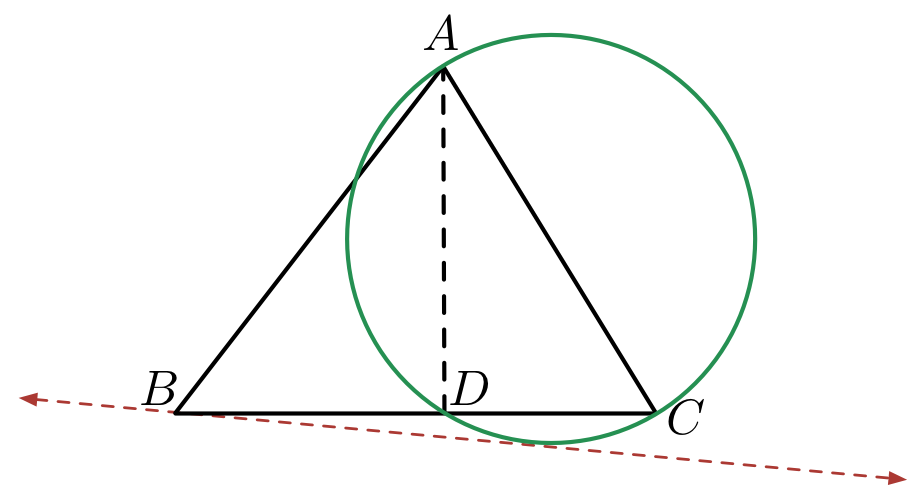# Find the length of the tangent

Geometry Level 5ABC is an acute angle triangle with side AC=10cm. A perpendicular is drawn from point A on side BC that cuts the side BC at point D. Length of AD=6cm. $\angle BAD=30°$. Then two tangents are drawn from points B and C to the circumcircle of ΔACD &ΔABD respectively. Consider those two tangents are BT&CS respectively. Then find the ratio of $\frac{BT×CS}{BC}$

×